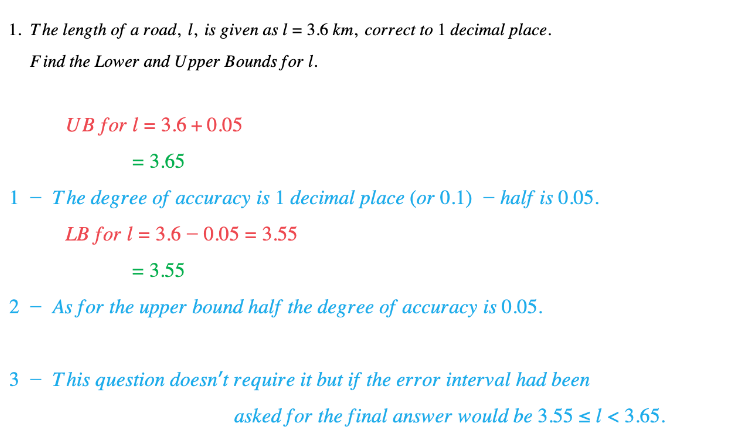# Edexcel IGCSE Maths复习笔记 1.12.1 Bounds & Error Intervals - Basics

Edexcel IGCSE Maths复习笔记 1.12.1 Bounds & Error Intervals - Basics

#### What are bounds?

• Bounds are the smallest – the Lower Bound (LB) – and largest – the Upper Bound (UB) – numbers that a rounded number can lie between

#### How do we find bounds?

• The basic rule is “Half Up, Half Down”
• More formally:
• UPPER BOUND – add on half the degree of accuracy
• LOWER BOUND – take off half the degree of accuracy
• ERROR INTERVAL: LB ≤ x < UB

• Note:It is very tempting to think that the Upper Bound should end in a 9, or 99, etc. but if you look at the Error Interval – LB ≤ x < UB – it does NOT INCLUDE the Upper Bound so all is well

Worked Example